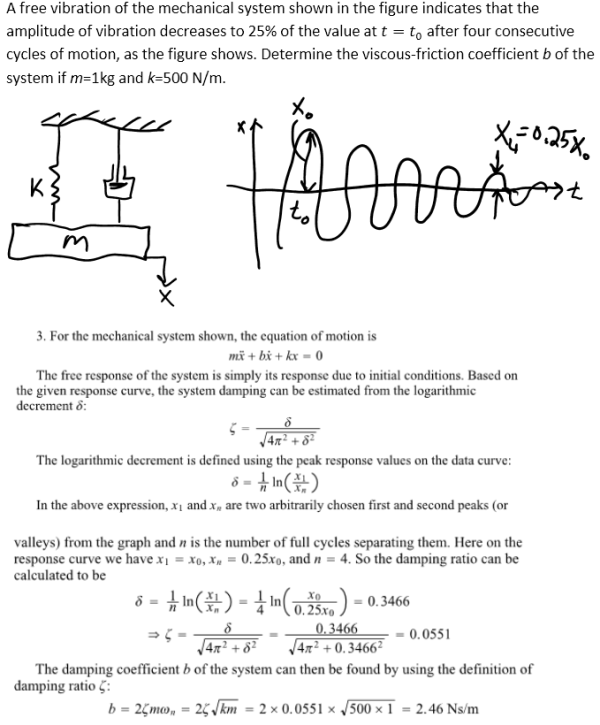A free vibration of the mechanical system shown in the figure indicates that the amplitude of vibration decreases to 25% of the value at t=t0 after four consecutive cycles of motion, as the figure shows. Determine the viscous-friction coefficient b of the system if m=1 kg and k=500 N/m.A free vibration of the mechanical system shown in the figure indicates that the amplitude of vibration decreases to 25% of the value at t=t0 after four consecutive cycles of motion, as the figure shows. Determine the viscous-friction coefficient b of the system if m=1 kg and k=500 N/m.

System Dynamics Page 2 dynamics dynamics dynamics dynamics dynamics dynamics dynamics System dynamics Page 3